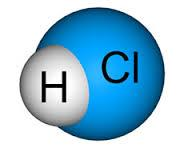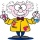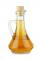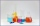# Hydrochloric acid

Determine the concentration of which must have a solution of hydrochloric acid that mixing 10 l of the solution with 8 liters of 26% solution to get the solution with a concentration of 50%?

x =  69.2 %

### Step-by-step explanation:

10•x/100+8•0.26=0.50•(10+8)

10x = 692

x = 346/5 = 69.2

Our simple equation calculator calculates it.Did you find an error or inaccuracy? Feel free to write us. Thank you!Tips to related online calculators
Looking for a statistical calculator?
Tip: Our volume units converter will help you with the conversion of volume units.

## Related math problems and questions:

• Mixture 2How many liters of water must be added to 7 liters of a 20% solution to obtain a 10% solution?
• Acid solutionBy adding 250 grams of a 96% sulfuric acid solution to its 3% solution, we changed its initial concentration to 25%. How many grams of 3% of the acid was used for dilution?
• Alcohol6l 48% alcohol, how much 52% alcohol must be added to give 50% alcohol?
• ConcentrationHow many grams of 65% and 50% acid is to be mixed to give 240 g acid with a concentration of 60%?
• CuSO4 mixtureHow many grams of solid CuSO4 we have to add to 450g of 15% CuSO4 solution to produce a 25% solution?
• GramsHow many grams of 5% solution do we need to add to 100g of 50% solution to get a 20% solution?
• Solutions, mixturesWe have 2 liters of 20% solution available. How much 70% solution do we need to add to it to get a 30% solution?
• FertilizerI have 4 liters of 10% fertilizer. How much water do I need to add to have a 2% solution?
• ConcentrationHow 0.5 liters of 8% vinegar diluted to a concentration of 20 hundredths % of vinegar? How many liters of water must be poured?
• SolutionsHow much 60% solution and how much 35% solution is needed to create 100 l of 40% solution?
• Solutions, mixturesHow many liters of 70% solution must we add to 5 liters of 30% solution to give us a 60% solution?
• PharmacyAt the pharmacy are in one container 20% solution in the second 50% solution of disinfectant. They need to prepare 4 L of 48-percent solution. What amount of solution from each container is needed to mix?
• Vinegar 2How many percentages of vinegar solution will we get if we mix 3.5 liters of 5.8% and 5 liters of 7.6% vinegar?
• Acid evaporationHow many kilograms of water do we have to evaporate from 100 kg of 32% acid to make it 80% concentration?
• Alcohol solutionsWe have to produce 2 liters of 60% alcohol from 55% and 80%. How many of which ones will we use in the solution?
• PeroxideHow many ml should we pour 30% of peroxide (H2O2) into 100ml H2O to give a 20% solution?
• Alcohol mixingHow much 64% of the alcohol must be poured into 6 liters of 85% alcohol to produce 79% alcohol?